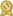Found 3800 calculus tutors – page 10 of 380.

Fort Smith 72908 · \$40/hour · teaches Bible Studies - Calculus - Elementary Math -

I am a licensed secondary math teacher. I am currently teaching calculus, pre-calc, and geometry at Union Christian Academy. I also have a master's in ESL besides a master's in business administration.certified

Huntersville 28078 · \$50/hour · teaches C - Calculus - Chess - Geometry - German - GMAT

I tutor SAT, ACT, GRE , GMAT test prep, math 1-3, AFM, pre-calculus, high school calculus, prealgebra, algebra, trigonometry, geometry, German, and some other subjects. My Qualifications: I have been...certified

Louisville 40205 · \$50/hour · teaches ACT Math - Algebra 1 - Algebra 2 - Geometry -

I am a former math teacher and an expert in all levels of mathematics through AP Calculus. I have a Master's degree (M.A.T. in Secondary Mathematics) from the University of Louisville.certified

Keller 76244 · \$50/hour · teaches Algebra 2 - Calculus - Elementary Math - Geometry -

I am very passionate when it comes to math and science. My strongest strength is math. My favorite math is algebra, but I also have a deep understanding of statistic, geometry, trigonometry, pre-algebra, pre-calculus...certified

Carlsbad 92010 · \$60/hour · teaches Algebra 2 - Calculus - Elementary Math - GED -

My main area of expertise is mathematics but I am available for tutoring physics as well. I have a degree in math. I was top of my class in calculus 1, calculus 3 and linear algebra.certified

Long Beach 90803 · \$60/hour · teaches Astronomy - Calculus - Finance - Geometry -

Hello! My name is David, and I hope to be the tutor you are looking for. I have almost 10 years of tutoring experience in all math subjects, including Algebra, Geometry, Trigonometry, Pre-Calculus, Calculus...certified

Hilton Head Island 29928 · \$45/hour · teaches Algebra 2 - Calculus - Discrete Math -

After completing a Ph.D. in mathematics, I began a 30-year teaching career at the university level. During this time, I taught courses at all levels of mathematics. I enjoy teaching calculus...certified

Saugerties 12477 · \$40/hour · teaches ASVAB - Calculus - Geometry - Prealgebra -

Greetings, I graduated from SUNY New Paltz with a degree in secondary education for mathematics. I have taken several upper level math courses that include: Calculus 1-3, Introduction to Proofs, Axiomatic Geometry...certified

Olathe 66062 · \$30/hour · teaches C++ - Calculus - Computer Programming - Elementary

...precalculus and calculus. This current year of 2017-2018 I am teaching the following subjects: two high school students calculus, one high school student precalculus, one middle school student algecertified

Temple City 91780 · \$55/hour · teaches Algebra 2 - Calculus - Elementary (K-6th) -

I teach basic elementary math, Pre-Algebra, Algebra, Geometry, Trigonometry, Pre-Calculus, Calculus and SAT Math. My specialty is in Algebra 1, Algebcertified